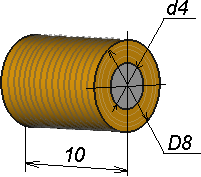QuickField

A new approach to field modelling
 Language: Global English Deutch Espanol Francais Italiano Danmark Ceske Chinese Pycckuü

>> >>

# Capacitor discharge through coil (RL-circuit). Co-simulation with Ngspice

Charged capacitor is connected to the coil. Coil is represented by inductor L1 connected in series with resistor R1.

Problem type:
Axisymmetric problem of DC magnetics

Geometry:Electric circuit schematics:Given:
Capacitor value C1 = 100 uF
Coil number of turns n = 500
Coil resistance value R1 = 4.5 Ohm
Core permeability μ - B(H) curve.

Calculate transient current in the electric circuit.

Solution:
VBA program (Microsoft Excel macros) generates a series of short time steps, with calls to QuickField and Ngspice at each step.
For given current value QuickField analyses the field distribution and the core saturation, and calculates the corresponding coil inductance value (L1). Circuit parameters are then written to a text file. Then Ngspice analyses the discharge process at this time step according to the circuit parameters read from the file (and taking into account the L1 value obtained in QuickField). The current value calculated in Ngspice at the end of the current time step is then submitted to QuickField for the next time step simulation.
Duration and number of the time steps are defined in the VBA program user interface arranged as an Excel table.Result:

Capacitor voltage and currentCoil inductance as a function of current• Video:Watch on YouTube.

• Download simulation files (files may be viewed using any QuickField Edition).